# Faber polynomials

(diff) ← Older revision | Latest revision (diff) | Newer revision → (diff)

A classical basis system that serves to represent analytic functions in a complex domain. Suppose that the complement of a bounded continuumcontaining more than one point is a simply-connected domainof the extended complex plane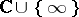, and that the function,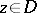, is the conformal univalent mapping ofonto the domain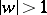under the conditions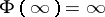and. Then the Faber polynomials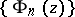can be defined as the sums of the terms of non-negative degree inin the Laurent expansions of the functions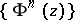in a neighbourhood of the point. The Faber polynomials forcan also be defined as the coefficients in the expansion(1)

where the functionis the inverse of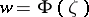. Ifis the disc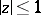, then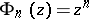. In the case whenis the segment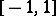, the Faber polynomials are the Chebyshev polynomials of the first kind. These polynomials were introduced by G. Faber .

Ifis the closure of a simply-connected domainbounded by a rectifiable Jordan curve, and the functionis analytic in, continuous in the closed domainand has bounded variation on, then it can be expanded inin a Faber series(2)

that converges uniformly inside, that is, on every closed subset of, where the coefficients in the expansion are defined by the formula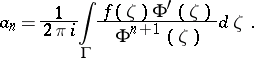The Faber series (2) converges uniformly in the closed domain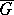if, for example,has a continuously-turning tangent the angle of inclination to the real axis of which, as a function of the arc length, satisfies a Lipschitz condition. Under the same condition on, the Lebesgue inequalityholds for every functionthat is analytic inand continuous in, where the constant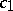is independent ofand, and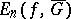is the best uniform approximation toinby polynomials of degree not exceeding.

One can introduce a weight function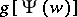in the numerator of the left-hand side of (1), whereis analytic in, is different from zero and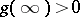. Then the coefficients of the expansion (1) are called generalized Faber polynomials.

How to Cite This Entry:
Faber polynomials. Encyclopedia of Mathematics. URL: http://encyclopediaofmath.org/index.php?title=Faber_polynomials&oldid=17377
This article was adapted from an original article by P.K. Suetin (originator), which appeared in Encyclopedia of Mathematics - ISBN 1402006098. See original article